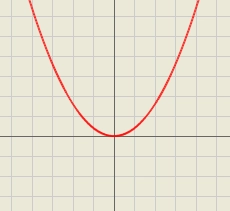# Suppose 10

Suppose 4+7i is a solution of 5z2+Az+B=0, where A, B∈R. Find A and B.

A =  -40
B =  325

### Step-by-step explanation:Did you find an error or inaccuracy? Feel free to write us. Thank you!

Tips for related online calculators
Are you looking for help with calculating roots of a quadratic equation?
Do you have a linear equation or system of equations and looking for its solution? Or do you have a quadratic equation?
Try our complex numbers calculator.

#### Grade of the word problem:

We encourage you to watch this tutorial video on this math problem: